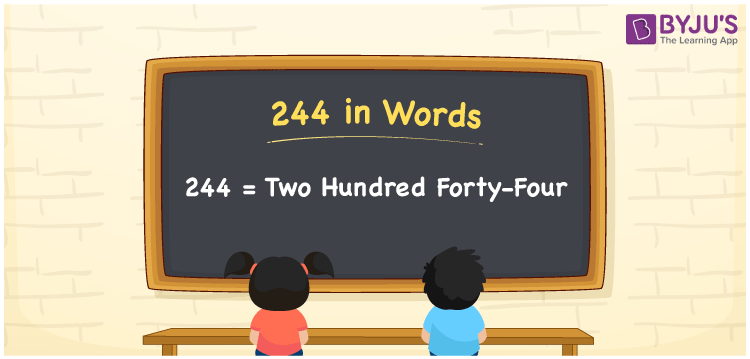# 244 in Words

Two hundred forty-four is the number name of the numeral 244. Using the ones, tens and hundreds places of a number, we can easily convert the number 244 into words. As we know, the number 244 appears between 243 and 245. This article discusses the procedure of writing the number 244 in words using the place value system in detail.

 244 in Words: Two Hundred Forty-four. Two Hundred Forty-four in Numerical Form: 244.

## 244 in English Words## How to Write 244 in Words?

The following table represents the place values of 244:

 Hundreds Tens Ones 2 4 4

The expanded form of 244 is as follows:

= 2 × Hundred + 4 × Ten + 4 × One

= 2 × 100 + 4 × 10 + 4 × 1

= 200 + 40 + 4

= 244

= Two hundred forty-four

Hence, 244 in words is two hundred forty-four.

244 in words – Two hundred forty-four

Is 244 an odd number? – No

Is 244 an even number? – Yes

Is 244 a perfect square number? – No

Is 244 a perfect cube number? – No

Is 244 a prime number? – No

Is 244 a composite number? – Yes

## Frequently Asked Questions on 244 in Words

Q1

### How to spell 244?

244 in words is two hundred forty-four.

Q2

### Simplify 200 + 44, and express it in words.

Simplifying 200 + 44, we get 244. Hence, 244 in words is two hundred forty-four.

Q3

### Is 244 an odd number?

No, 244 is not an odd number.